Home

# An improved model of near-inertial wave dynamics

## Abstract

The YBJ equation (Young & Ben Jelloul, J. Marine Res., vol. 55, 1997, pp. 735–766) provides a phase-averaged description of the propagation of near-inertial waves (NIWs) through a geostrophic flow. YBJ is obtained via an asymptotic expansion based on the limit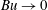$\mathit{Bu}\rightarrow 0$ , where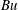$\mathit{Bu}$ is the Burger number of the NIWs. Here we develop an improved version, the YBJ+ equation. In common with an earlier improvement proposed by Thomas, Smith & Bühler (J. Fluid Mech., vol. 817, 2017, pp. 406–438), YBJ+ has a dispersion relation that is second-order accurate in$\mathit{Bu}$ . (YBJ is first-order accurate.) Thus both improvements have the same formal justification. But the dispersion relation of YBJ+ is a Padé approximant to the exact dispersion relation and with$\mathit{Bu}$ of order unity this is significantly more accurate than the power-series approximation of Thomas et al. (2017). Moreover, in the limit of high horizontal wavenumber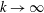$k\rightarrow \infty$ , the wave frequency of YBJ+ asymptotes to twice the inertial frequency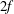$2f$ . This enables solution of YBJ+ with explicit time-stepping schemes using a time step determined by stable integration of oscillations with frequency$2f$ . Other phase-averaged equations have dispersion relations with frequency increasing as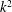$k^{2}$ (YBJ) or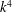$k^{4}$ (Thomas et al. 2017): in these cases stable integration with an explicit scheme becomes impractical with increasing horizontal resolution. The YBJ+ equation is tested by comparing its numerical solutions with those of the Boussinesq and YBJ equations. In virtually all cases, YBJ+ is more accurate than YBJ. The error, however, does not go rapidly to zero as the Burger number characterizing the initial condition is reduced: advection and refraction by geostrophic eddies reduces in the initial length scale of NIWs so that$\mathit{Bu}$ increases with time. This increase, if unchecked, would destroy the approximation. We show, however, that dispersion limits the damage by confining most of the wave energy to low$\mathit{Bu}$ . In other words, advection and refraction by geostrophic flows does not result in a strong transfer of initially near-inertial energy out of the near-inertial frequency band.

## References

Hide All
MathJax
MathJax is a JavaScript display engine for mathematics. For more information see http://www.mathjax.org.

# An improved model of near-inertial wave dynamics

## Metrics

### Full text viewsFull text views reflects the number of PDF downloads, PDFs sent to Google Drive, Dropbox and Kindle and HTML full text views.

Total number of HTML views: 0
Total number of PDF views: 0 *Loading metrics...

### Abstract viewsAbstract views reflect the number of visits to the article landing page.

Total abstract views: 0 *Loading metrics...

* Views captured on Cambridge Core between <date>. This data will be updated every 24 hours.

Usage data cannot currently be displayed